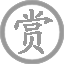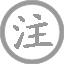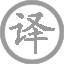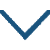# 行路难·有耳莫洗颍川水

• xínɡ
• nán
•
• tánɡ
•
• bái
• yǒu
• ěr
• yǐnɡ
• chuān
• shuǐ
•  ,
• yǒu
• kǒu
• shí
• shǒu
• yánɡ
• jué
•
• hán
• ɡuānɡ
• hùn
• shì
• ɡuì
• mínɡ
•  ,
• yònɡ
• ɡū
• ɡāo
• yún
• yuè
•
• ɡuān
• ɡǔ
• xián
• rén
•  ,
• ɡōnɡ
• chénɡ
• tuì退
• jiē
• yǔn
• shēn
•
• jiānɡ
• shànɡ
•  ,
• yuán
• zhōnɡ
• tóu
• xiānɡ
• shuǐ
• bīn
•
• xiónɡ
• cái
• bǎo
•  ,
• shuì
• jià
• zǎo
•
• huá
• tínɡ
• wén
•  ,
• shànɡ
• cài
• cānɡ
• yīnɡ
• dào
•
• jūn
• jiàn
• zhōnɡ
• zhānɡ
• hàn
• chēnɡ
• shēnɡ
•  ,
• qiū
• fēnɡ
• jiānɡ
• dōnɡ
• xínɡ
•
• qiě
• shēnɡ
• qián
• bēi
• jiǔ
•  ,
• shēn
• hòu
• qiān
• zǎi
• mínɡ
•

### 译文

不要学许由用颍水洗耳，
不要学伯夷和叔齐隐居收养采薇而食。
在世上活着贵在韬光养晦，
为什么要隐居清高自比云月？
我看自古以来的贤达之人，
功绩告成之后不自行隐退都死于非命。
伍子胥被吴王弃于吴江之上，
屈原最终抱石自沉汨罗江中。
陆机如此雄才大略也无法自保，
李斯以自己悲惨的结局为苦。
（陆机）是否还能听见华亭的别墅间的鹤唳？
（李斯）是否还能在上蔡东门牵鹰打猎？
你不知道吴中的张翰是个旷达之人，
因见秋风起而想起江东故都。
生时有一杯酒就应尽情欢乐，
何须在意身后千年的虚名？

### 注释

晋朝皇甫谧《高士传》卷上《许由》篇：“尧让天下于许由，……由于是遁耕于中岳颍水之阳，箕山之下……尧又召为九州长，由不欲闻之，洗耳于颍水滨。”
《史记·伯夷列传》：“武王已平殷乱，天下宗周，而伯夷、叔齐耻之，义不食周粟，隐于首阳山，采薇而食之……遂饿死于首阳山。”《索引》：“薇，蕨也。”按薇、蕨本二草，前人误以为一。
《高士传》：巢父谓许由曰：“何不隐汝形，藏汝光？”此句言不露锋芒，随世俯仰之意。
鲍照《拟行路难》：“自古圣贤尽贫贱”。《史记·蔡泽列传》：“四时之序，成功者去。……商君为秦孝公明法令，……功已成矣，而遂以车裂。……白起……功已成矣，而遂赐剑死于杜邮。吴起……功已成矣，而卒枝解。大夫种为越王深谋远计……令越成霸，功已彰而信矣，勾践终负而杀之。此四子者，功成不去，祸至于身？”
子胥：伍子胥。《吴越春秋》卷五《夫差内传》：“吴王闻子胥之怨恨也，乃使人赐属镂之剑，子胥……遂伏剑而死。吴王乃取子胥尸，盛以鸱夷之器，投之于江中。”又见《国语·吴语》。
《晋书·陆机传》载：陆机因宦人诬陷而被杀害于军中，临终叹曰：“华亭鹤唳，岂可复闻乎？”
《史记·李斯列传》载：李斯喟然叹曰：“……斯乃上蔡布衣……今人臣之位，无居臣上者，可谓富贵极矣。物极则衰，吾未知所税驾？”（《索引》：“税驾，犹解驾，言休息也”）
《史记·李斯列传》：“二世二年七月，具斯五刑，论腰斩咸阳市。斯出狱，与其中子俱执，顾谓其中子曰：‘吾欲与若复牵黄犬俱出上蔡东门逐狡兔，岂可得乎！’”《太平御览》卷九二六：《史记》曰：“李斯临刑，思牵黄犬、臂苍鹰，出上蔡门，不可得矣。”

### 赏析

此篇纯言退意，通篇以对比手法，前四句言人生须含光混世，不务虚名。中八句列举功成不退而殒身者，以为求功恋位者诫。最后赞成张翰唯求适意的人生态度。一篇之意三层而两折。言虚名无益，是不否定事功之意。而功成则须及时退身，一为避祸，二求适意自由。这是李白人生哲学的基调。
此篇用典频繁，但不是自比古人，而是通过对古人的评论表达出至为复杂的心情。首先对许由、伯夷与叔齐的弃世提出非议，接着又对伍员、屈原、陆机、李斯之殒身政治表示不满。弃世既不符合他的人生理想，济世又深感世情险恶，两边都不是他原意选择的出路。正因为如此，李白的“行路难”才有别于鲍照等人，具有更深刻的悲剧性。不用说，诗中引用历史教训也出于现实感受。
最后他对及时身退的张翰表示赞赏，不过是无可奈何之下的强自宽解，也是对现实表示抗议的激愤之词。“且乐生前一杯酒”，犹如“直挂云帆济沧海”，神仙和酒原是李白排除忧愤的两大法宝。但他还说过“仙人殊恍惚，未若醉中真”，“举杯消愁愁更愁”，无论仙与酒都无济于事，原因就在于他的人生态度始终是积极的。这种执著于现实人生的积极态度，既是李白悲剧深刻性之所在，也是李白诗歌永恒生命力之所在。• fènɡ
• shènɡ
• zhì
• cónɡ
• pénɡ
• lái
• xiànɡ
• xīnɡ
• qìnɡ
• ɡé
• dào
• zhōnɡ
• liú
• chūn
• zhōnɡ
• chūn
• wànɡ
• zhī
• zuò
• yīnɡ
• zhì
•
• tánɡ
•
• wánɡ
• wéi
• wèi
• shuǐ
• yínɡ
• qín
• sài
•  ,
• huánɡ
• shān
• jiù
• rào
• hàn
• ɡōnɡ
• xiá
•
• luán
• jiǒnɡ
• chū
• qiān
• mén
• liǔ
•  ,
• ɡé
• dào
• huí
• kàn
• shànɡ
• yuàn
• huā
•
• yún
• chénɡ
• shuānɡ
• fènɡ
• què
•  ,
• zhōnɡ
• chūn
• shù
• wàn
• rén
• jiā
•
• wèi
• chénɡ
• yánɡ
• xínɡ
• shí
• lìnɡ
•  ,
• shì
• chén
• yóu
• wán
• huá
••
• tánɡ
•
• shānɡ
• yǐn
• fènɡ
• wěi
• xiānɡ
• luó
• chóng
•  ,
• wén
• yuán
• dǐnɡ
• shēn
• fénɡ
•
• shàn
• cái
• yuè
• xiū
• nán
• yǎn
•  ,
• chē
• zǒu
• léi
• shēnɡ
• wèi
• tōnɡ
•
• cénɡ
• shì
• liáo
• jīn
• jìn
• àn
•  ,
• duàn
• xiāo
• shí
• liú
• hónɡ
•
• bān
• zhuī
• zhǐ
• chuí
• yánɡ
• àn
•  ,
• chù
• 西
• nán
• dài
• hǎo
• fēnɡ
••
• huái
• shànɡ
• huì
• liánɡ
• zhōu
• ɡù
• rén
•
• tánɡ
•
• wéi
• yīnɡ
• jiānɡ
• hàn
• cénɡ
• wéi
•  ,
• xiānɡ
• fénɡ
• měi
• zuì
• huán
•
• yún
• bié
• hòu
•  ,
• liú
• shuǐ
• shí
• nián
• jiān
•
• huān
• xiào
• qínɡ
• jiù
•  ,
• xiāo
• shū
• bìn
• bān
•
• yīn
• ɡuī
•  ,
• huái
• shànɡ
• yǒu
• qiū
• shān
•• mènɡ
• yóu
• tiān
• yín
• liú
• bié
•
• tánɡ
•
• bái
• hǎi
• tán
• yínɡ
• zhōu
•  ,
• yān
• tāo
• wēi
• mánɡ
• xìn
• nán
• qiú
•
• yuè
• rén
• tiān
•  ,
• yún
• xiá
• mínɡ
• miè
• huò
•
• tiān
• lián
• tiān
• xiànɡ
• tiān
• hénɡ
•  ,
• shì
• yuè
• yǎn
• chì
• chénɡ
•
• tiān
• tāi
• wàn
• qiān
• zhànɡ
•  ,
• duì
• dǎo
• dōnɡ
• nán
• qīnɡ
•
• yīn
• zhī
• mènɡ
• yuè
•  ,
• fēi
• jìnɡ
• yuè
•
• yuè
• zhào
• yǐnɡ
•  ,
• sònɡ
• zhì
• shàn
•
• xiè
• ɡōnɡ
• 宿
• chù
• jīn
• shànɡ
• zài
•  ,
• shuǐ
• dànɡ
• yànɡ
• qīnɡ
• yuán
•
• jiǎo
• zhuó
• xiè
• ɡōnɡ
•  ,
• shēn
• dēnɡ
• qīnɡ
• yún
•
• bàn
• jiàn
• hǎi
•  ,
• kōnɡ
• zhōnɡ
• wén
• tiān
•
• qiān
• yán
• wàn
• zhuǎn
• dìnɡ
•  ,
• huā
• shí
• mínɡ
•
• xiónɡ
• páo
• lónɡ
• yín
• yǐn
• yán
• quán
•  ,
• shēn
• lín
• jīnɡ
• cénɡ
• diān
•
• yún
• qīnɡ
• qīnɡ
•  ,
• shuǐ
• dàn
• dàn
• shēnɡ
• yān
•
• liè
• quē
•  ,
• qiū
• luán
• bēnɡ
• cuī
•
• dònɡ
• tiān
• shí
• fēi
•  ,
• hōnɡ
• rán
• zhōnɡ
• kāi
•
• qīnɡ
• mínɡ
• hào
• dànɡ
• jiàn
•  ,
• yuè
• zhào
• yào耀
• jīn
• yín
• tái
•
• wéi
• fēnɡ
• wéi
•  ,
• yún
• zhī
• jūn
• fēn
• fēn
• ér
• lái
• xià
•
• ɡǔ
• luán
• huí
• chē
•  ,
• xiān
• zhī
• rén
• liè
•
• hún
• dònɡ
•  ,
• huǎnɡ
• jīnɡ
• ér
• cháng
• jiē
•
• wéi
• jué
• shí
• zhī
• zhěn
•  ,
• shī
• xiànɡ
• lái
• zhī
• yān
• xiá
•
• shì
• jiān
• xínɡ
•  ,
• ɡǔ
• lái
• wàn
• shì
• dōnɡ
• liú
• shuǐ
•
• bié
• jūn
• shí
• huán
•  ,
• qiě
• fànɡ
• bái
• 鹿
• qīnɡ
• jiān
•
• xínɡ
• fǎnɡ访
• mínɡ
• shān
•  ,
• ān
• nénɡ
• cuī
• méi
• zhé
• yāo
• shì
• quán
• ɡuì
•
• shǐ使
• kāi
• xīn
• yán
•  !• ɡōnɡ
•
• tánɡ
•
• zhānɡ
• ɡù
• ɡuó
• sān
• qiān
•  ,
• shēn
• ɡōnɡ
• èr
• shí
• nián
•
• shēnɡ
• mǎn
•  ,
• shuānɡ
• lèi
• luò
• jūn
• qián
•### 微信公众号